## 时间序列分析:AR(p),MA(q)

1.1 数学期望函数、方差函数1.2 自相关函数与平稳随机过程ρxy=0时说明随机变量X，Y没有线性相关性，ρxy接近1时两者有较强正线性相关性，ρxy接近-1时两者有较强负线性相关性，需要强调的是，协方差系数ρxy等于0只能说明两个随机变量没有线性相关性，并不代表X、Y没有诸如这样的非线性相关性。随机过程也有类似协方差的概念，由于随机过程各个样本函数都源自同一个随机变量，所谓的相关性都是与自身比较，在任意两个时刻t1、t2,随机过程的自相关函数定义为：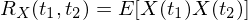(1.1)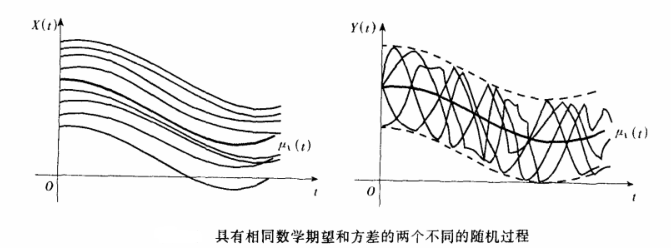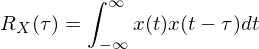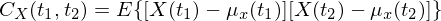(1.2)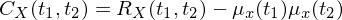(1.3)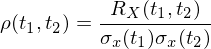(1.4)

随机过程离散化表示方式是{X(t),t∈T},T=0,±1,±2,±3,±4,...,如果随机过程{X(t),t∈T}满足以下三个条件：

1、数学期望函数是常数

2、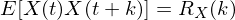自相关函数仅与间隔k有关，与时刻t无关

3、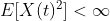原点二阶矩存在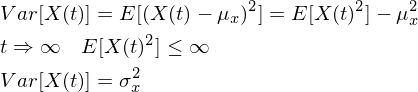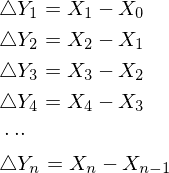2.1  AR(p)平稳性判断、自相关系数、偏相关系数

AR(p)模型表达式为：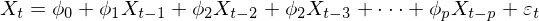（2.1）

(2.1)表明Xt与前面p项随机变量有关，εt表示数学期望为0、标准差为σε随机误差，且任意两个时刻的误差相互独立：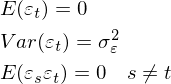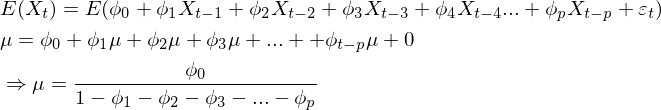（2.1.1）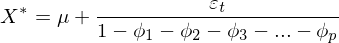(2.2)

(2.2)式表明如果一个形如AR(p)的随机过程为平稳随机过程，那么其稳定后时间序列将围绕常数μ上下有限波动，到目前为止所有的结论都是基于假设该随机过程为平稳随机过程， AR(p)表达式为线性差分方程，线性差分方程的解等于通解加上特解，特解是一个常数，不妨就把稳定解X*作为特解，而(2.1)的通解是下面线性齐次方程的解。（2.3）,上式可写为：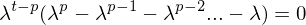（2.4）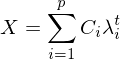(Ci为常数)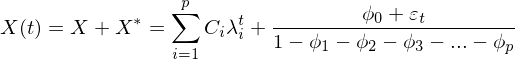（2.5）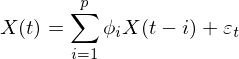（2.6）(2.6.1)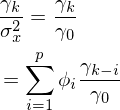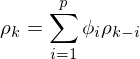(2.7)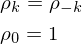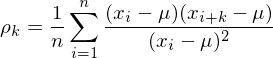(2.8)

```import numpy as np
from statsmodels.stats.diagnostic import acorr_ljungbox
from statsmodels.tsa.stattools import  acf,pacf
def generateerr(fn=np.random.randn):
err = fn(1, 1)[0, 0]
return err
def genardata(num):
y=[]
y.append(0)
#y.append(0)
for i  in range(num):
err=generateerr()
y.append( y[-1]*0.65  +err)
return np.array(y)
#计算自相关系数
def acfvalues(TimeSeries):
Xt,Xt_k,AutoCovariance  = [],[],[]
avg = np.mean(TimeSeries)
i = 1
while i < len(TimeSeries):
t,t_k = TimeSeries[i::],TimeSeries[:-i:]
Xt.append(t)
Xt_k.append(t_k)
i += 1
k = 0
result_temp0 = 0
# 首先求γ0的值
while k < len(TimeSeries):
result_temp0 = result_temp0 + pow((TimeSeries[k] - avg), 2)
k += 1
AutoCovariance.append(result_temp0 / len(TimeSeries))
# 然后计算分子
p = 0
q = 0
while p < len(Xt):
q = 0
result_temp1 = 0
while q < len(Xt[p]):
result_temp1 = result_temp1 + (Xt[p][q] - avg) * (Xt_k[p][q] - avg)
q += 1
AutoCovariance.append(result_temp1 / len(TimeSeries))
p += 1
#以上为自相关函数计算过程，除以γ0得到自相关系数
pks = [ t/AutoCovariance for t in AutoCovariance]
return pks

if __name__=='__main__':
N=1000
y=genardata(N)
# 是否为非平稳序列
print('平稳随机过程判断:')
print(pvalues)
# 是否为白噪音
pvalues = acorr_ljungbox(y, lags=[1, 2, 3, 4, 5 ])
print('白噪音判断:')
print(pvalues)
print('实现自相关系数计算:')
print(acfvalues(y)[0:10])
acfs_stattools  = acf(y,nlags=10 )
print('自相关系数(stattools):')
print(acfs_stattools[:])```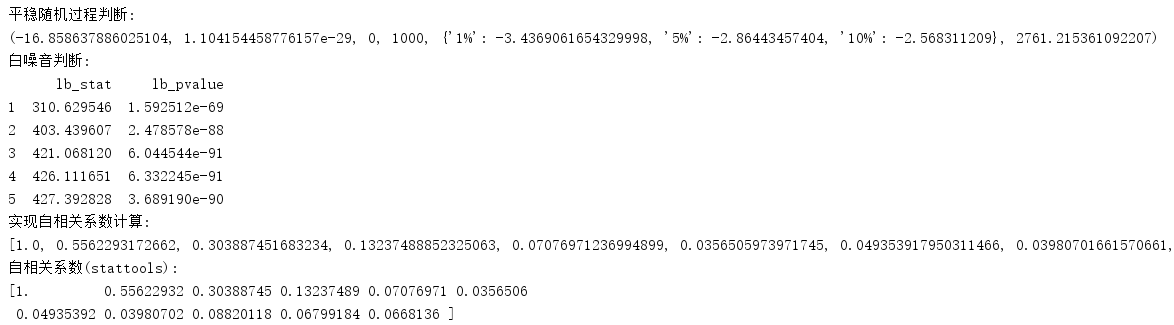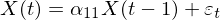α11为1阶偏自相关系数，上式两边同乘以X(t-1)取数学期望，并除以方差后得：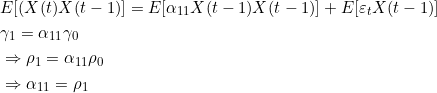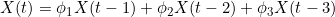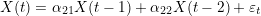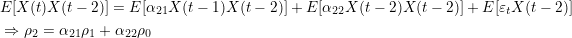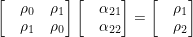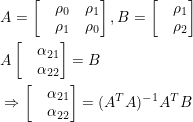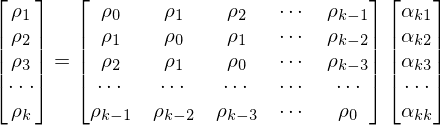(2.9)

2.2  MA(q)统计数学特征

MA(q)的表达形式为：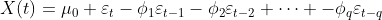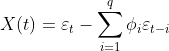(2.2.1)

MA(q)数学期望函数、方差函数：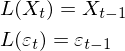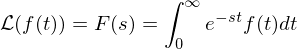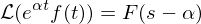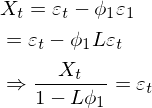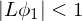时，上式可以写为幂级数形式：（2.2.2）

MA(1)可以表示成AR(p)无穷级数的形式,MA(q)用归纳法可以得到相同的结论，利用Yule-Walker方程组解MA偏自相关系数可以到无穷阶，所以MA(q)的偏自相关系数呈现出拖尾性，根据公式1.1MA(q)k阶自相关函数定义为：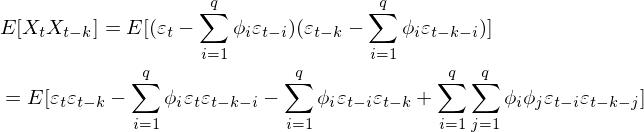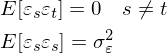k=0时，k阶自相关函数等于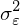a) 1<=k<=q 时，k阶自相关函数等于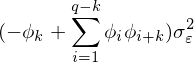b) k>q时，k阶自相关函数等于0。

c) 自相关函数除以方差得到MA(q)的k阶自相关系数公式：(2.2.3)

k>q时相关系数等于0说明MA(q)自相关系数具有q阶截尾性，观察(2.2.3)可以发现当q>=2时，给定一个自相关系数ρk,可通过一组q元q次方程组求解参数,方程有多个根，也就是说对于同一个自相关系数有多个不同MA(q)模型与之对应，如下面两个MA(2)模型：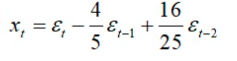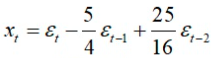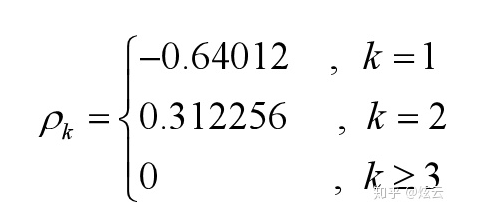X(t)在t趋近于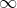后收敛于0，上式右边为差分方程，可设通解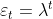，得到特征方程：（2.2.4）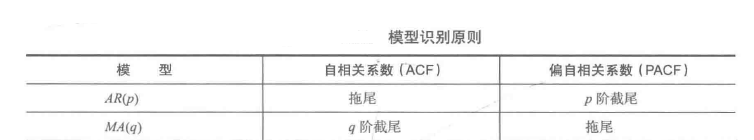3.1 回归法求解AR(p)参数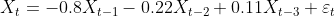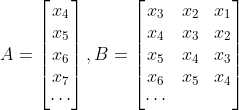-免费试读结束-

 上一篇  K-Means聚类算法 评论区